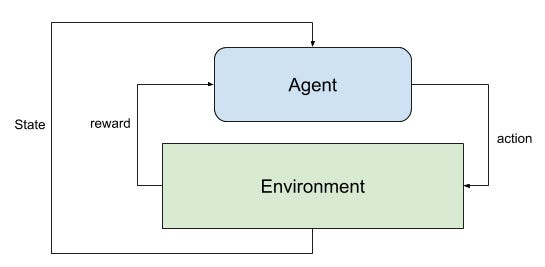03. Reinforcement Learning (Move 37): Markov Decision Processes

A summary of the concepts discussed in the third lecture for a reinforcement learning course from the School of AI called Move 37. The focus of this lecture was on Markov Decision Processes. See my previous post here for an introduction to the Bellman Equation lecture notes summary.

Markov Decision Process (MDP)

Markovian property:

The future state is independent of any previous states history given the current state and action. Therefore the current state encapsulates all that is needed to decide the future state when an input action is received. (E.g Chess Board)

Policy is the solution to a MDP and the objective is to find the optimal policy for a task that MDP is imposed.

MDP Concepts

State: Set of tokens that represent every condition that the agent can be in.

Model (Transition Model): Gives an action’s effect in a state. T(S,a,S’) defines a transition T where you start in state S and take an action ‘a’ to move to state S’. Stochastic actions (noisy, non-deterministic) define a probability P(S’|S,a) which represents the probability of reaching a state S’ if action ‘a’ is taken in state S.

Reward: A reward is a real-valued response to an action.

• R(S) indicates the reward for being in the state S.
• R(S,a) indicates the reward for being in a state S and taking an action ‘a’.
• R(S,a,S’) indicates the reward for being in a state S, taking an action ‘a’ and ending up in a state S’.

Policy: A policy is a solution to the MDP. A policy is a set of actions that are taken by the agent to reach a goal. A policy is denoted as π(s)

π* is called the optimal policy, which maximises the expected reward. For an MDP, there’s no end of the lifetime and you have to decide the end time.

Markov Decision Process (MDP) is a tuple(S,A,T,r,γ):

Rewards specify what the agent needs to achieve, not how to achieve it.

S = Set of observations. The agent observes the environment state as one item of this set.
A = Set of actions. The set of actions the agent can choose one from to interact with the environment.
T : P(s’ | s, a) Transition probability matrix. This models what next state s’ will be after the agent makes the action a while being in the current state s.

r : P(r | s, a) Reward model. Models what reward the agent will receive when it performs action a when it is in state s.

γ = discount factor. Value between 0 and 1 that represents the relative importance between immediate and future rewards.

The next summary will be on the reinforcement learning for sensor networks. If you would like post updates follow me here and/or on Twitter. Feedback and comments are also appreciated.

Source: Deep Learning on Medium